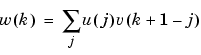MATLAB Function Referenceconv

Convolution and polynomial multiplication

Syntax

• ```w = conv(u,v)
```

Description

```w = conv(u,v) ``` convolves vectors `u` and `v`. Algebraically, convolution is the same operation as multiplying the polynomials whose coefficients are the elements of `u` and `v`.

Definition

Let `m` `=` `length(u)` and `n` `=` `length(v)`. Then `w` is the vector of length `m+n-1` whose `k`th element is

•The sum is over all the values of `j` which lead to legal subscripts for `u(j)` and `v(k+1-j)`, specifically `j` `=` `max(1,k+1-n)`: `min(k,m)`. When `m` `=` `n`, this gives

• ```w(1) = u(1)*v(1)
w(2) = u(1)*v(2)+u(2)*v(1)
w(3) = u(1)*v(3)+u(2)*v(2)+u(3)*v(1)
...
w(n) = u(1)*v(n)+u(2)*v(n-1)+ ... +u(n)*v(1)
...
w(2*n-1) = u(n)*v(n)
```

Algorithm

The convolution theorem says, roughly, that convolving two sequences is the same as multiplying their Fourier transforms. In order to make this precise, it is necessary to pad the two vectors with zeros and ignore roundoff error. Thus, if

• ```X = fft([x zeros(1,length(y)-1)])
```

and

• ```Y = fft([y zeros(1,length(x)-1)])
```

then `conv(x,y) = ifft(X.*Y)`

See Also

`conv2`, `convn`, `deconv`, `filter`

`convmtx` and `xcorr` in the Signal Processing Toolbox

© 1994-2005 The MathWorks, Inc.